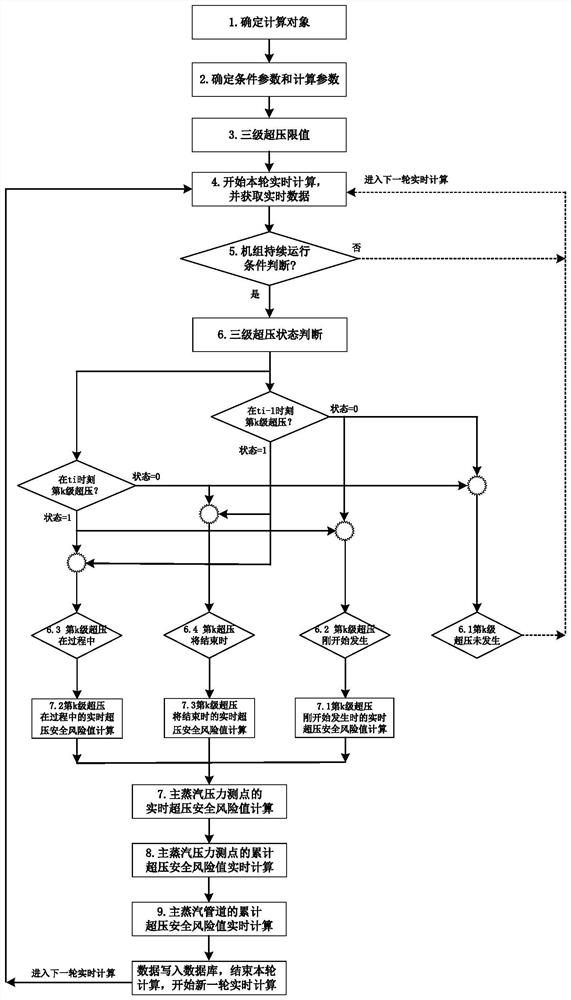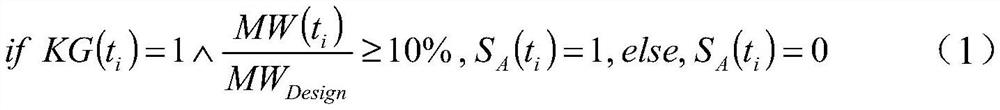# Real-time calculation method for three-level over-limit safety risk of pressure of main steam pipeline

## A technology of main steam pressure and pipeline pressure, which can be used in calculation, measurement of fluid pressure, complex mathematical operations, etc., and can solve problems such as real-time calculation methods that have not been reported.

Pending Publication Date: 2022-07-29
XIAN THERMAL POWER RES INST CO LTD +1
0 Cites 0 Cited by

## AI-Extracted Technical Summary

### Problems solved by technology

However, there is no report on the real-time calculation method for the safety risk o...
View more

## Abstract

The invention discloses a real-time calculation method for three-level over-limit safety risks of main steam pipeline pressure. The method comprises the following steps that whether kth-level over-pressure occurs or not is judged according to the kth-level over-pressure state SBk (ti-1) of each main steam pressure measuring point at the previous moment ti-1 and the kth-level over-pressure state SBk (ti) of each main steam pressure measuring point at the current moment ti; when the kth-level overpressure occurs, calculating the real-time overpressure safety risk value Rjk (ti) of each main steam pressure measuring point at the current moment ti according to the occurrence moment of the kth-level overpressure; according to the real-time overpressure safety risk value Rjk (ti) of each main steam pressure measuring point at the current moment ti, calculating an accumulated overpressure safety risk value Rj of each main steam pressure measuring point up to the calculation moment; and according to the accumulated overpressure safety risk value Rj of each main steam pressure measuring point up to the calculation moment, calculating the accumulated overpressure safety risk value R of the main steam pipeline up to the calculation moment. According to the method, the three-level overpressure safety risk of the pressure of the main steam pipeline is calculated in real time.

Application Domain

Data processing applicationsCircuit arrangements +2

Technology Topic

Structural engineeringMechanics +4

## Image

•••## Examples

• Experimental program(1)

### Example Embodiment

 In order to make those skilled in the art better understand the solutions of the present invention, the technical solutions in the embodiments of the present invention will be clearly and completely described below with reference to the accompanying drawings in the embodiments of the present invention. Obviously, the described embodiments are only The embodiments are part of the present invention, not all of the embodiments, and are not intended to limit the scope of the present disclosure. Also, in the following description, descriptions of well-known structures and techniques are omitted to avoid unnecessarily obscuring the concepts disclosed in the present invention. Based on the embodiments of the present invention, all other embodiments obtained by persons of ordinary skill in the art without creative efforts shall fall within the protection scope of the present invention.
 The accompanying drawings show a schematic structural diagram of an embodiment according to the disclosure of the present invention. The figures are not to scale, some details have been exaggerated for clarity, and some details may have been omitted. The shapes of various regions and layers shown in the figures and their relative sizes and positional relationships are only exemplary, and in practice, there may be deviations due to manufacturing tolerances or technical limitations, and those skilled in the art should Regions/layers with different shapes, sizes, relative positions can be additionally designed as desired.
 refer to figure 1 , the real-time calculation method of the safety risk of the third-level overrun safety risk of the main steam pipeline pressure according to the present invention comprises the following steps:
 1) Determine the calculation object
 According to the actual situation of the thermal power plant, the calculation object is the main steam pipeline on the A side or the B side of a single unit.
 2) Determine the condition parameters and calculation parameters
 Conditional parameters are: real-time grid-connected switch KG of the unit, real-time power generation MW of the unit and MW of the unit’s combined capacity Design;
 The calculation parameters are: main steam pressure MSP;
 When there are multiple main steam pressure measuring points on the main steam pipeline, they are distinguished by the j in the lower right corner, then the value of each measuring point is MSP j Participate in calculations independently.
 3) Determine the three-level overpressure limit
 The three-level overpressure limits are respectively the first-level overpressure limits LV I , secondary overpressure limit LV II and three-level overpressure limit LV III , where LV I II III.
 For the convenience of calculation, the overpressure of each level of the three-level overpressure is uniformly represented by the lower right subscript k, and the limit of the three-level overpressure is expressed as LV. k.
 4) Start calculation and get real-time data
 from the current time t i Start the calculation, obtain the condition parameters and calculation parameters from the database, and go to step 5);
 5) Judgment of the continuous operation condition of the unit
 Obtaining the condition parameters The real-time grid-connected switch of the unit and the real-time generating power of the unit at the current time t i The value of KG(t i ) and MW(t i ), then the current time t i The unit operating state S A (t i )for:

 Obtain the condition parameters of the unit's real-time grid-connected switch and the unit's real-time power generation at the previous time t i-1 The value of KG(t i-1 ) and MW(t i-1 ), where the previous time t i-1 The unit operating state S A (t i-1 )for:

 When (S A (t i )+S A (t i-1 )) ≥ 1, the unit continues to run, then go to step 6), otherwise, go to step 4);
 6) Judgment of three-level overpressure state
 Obtain each main steam pressure measurement point at the previous time t i-1 The numerical MSP of j (t i-1 ), calculate the time t before each main steam pressure measurement point i-1 The k-th stage overpressure state S Bk (t i-1 )for:
 if MSP j (t i-1 )≥LV k ,S Bk (t i-1 )=1, else, S Bk (t i-1 ) = 0 (3)
 Get each main steam pressure measurement point at the current time t i The numerical MSP of j (t i ), calculate each main steam pressure measuring point at the current time t i The k-th stage overpressure state S Bk (t i ):
 if MSP j (t i )≥LV k ,S Bk (t i )=1, else, S Bk (t i ) = 0 (4)
 When each main steam pressure measuring point is at the current time t i The k-th stage overpressure state S Bk (t i ) and the previous moment t i-1 The k-th stage overpressure state S Bk (t i-1 ) when the condition shown in formula (5) is satisfied, then the k-th overpressure does not occur, then go to step 4);
 if S Bk (t i-1 )=0∨S Bk (t i ) = 0 (5)
 When each main steam pressure measuring point is at the current time t i The k-th stage overpressure state S Bk (t i ) and the previous moment t i-1 The k-th stage overpressure state S Bk (t i-1 ) when the condition shown in formula (6) is satisfied, then the k-th overpressure just begins to occur, then go to step 71);
 if S Bk (t i-1 )=0∨S Bk (t i ) = 1 (6)
 When each main steam pressure measuring point is at the current time t i The k-th stage overpressure state S Bk (t i ) and the previous moment t i-1 The k-th stage overpressure state S Bk (t i-1 ) when the condition shown in formula (7) is satisfied, then the k-th level overpressure is in the process, then go to step 72);
 if S Bk (t i-1 )=1∨S Bk (t i ) = 1 (7)
 When each main steam pressure measuring point is at the current time t i The k-th stage overpressure state S Bk (t i ) and the previous moment t i-1 The k-th stage overpressure state S Bk (t i-1 ) when the condition shown in formula (8) is satisfied, then the k-th overpressure will end, then go to step 73):
 if S Bk (t i-1 )=1∨S Bk (t i ) = 0 (8)
7) Real-time overpressure safety risk value calculation of main steam pressure measuring points
 For different k-th overpressure states, according to each main steam pressure measurement point at the current time t i The numerical MSP of j (t i ) and at the previous time t i-1 The numerical MSP of j (t i-1 ) and the tertiary overpressure limit LV k , calculate the current time t i The real-time overpressure safety risk value of each main steam pressure measurement point, specifically:
 7-1) When the k-th overpressure of each main steam pressure measuring point just begins to occur, then the real-time overpressure safety risk value R of the main steam pressure measuring point jk (t i )for:

 7-2) When the k-th overpressure of each main steam pressure measuring point is in the process, then the real-time overpressure safety risk value R of the main steam pressure measuring point jk (t i )for:

 7-3) When the k-th overpressure of each main steam pressure measuring point will end, then the real-time overpressure safety risk value R of the main steam pressure measuring point jk (t i )for:

 8) Real-time calculation of cumulative overpressure safety risk value of main steam pressure measurement points
 According to each main steam pressure measuring point at the current time t i The real-time overpressure safety risk value R jk (t i ), calculate the cumulative overpressure safety risk value R of each main steam pressure measurement point up to the calculation time j for:

 where, t start Initial time calculated for the cumulative overpressure safety risk value, t now Calculates the current time for the cumulative overpressure safety risk value.
 9) Real-time calculation of the cumulative overpressure safety risk value of the main steam pipeline
 According to the accumulated overpressure safety risk value R of each main steam pressure measurement point up to the calculation time j , calculate the cumulative overpressure safety risk value R of the main steam pipeline as of the calculation time as:

 Among them, j indicates that the main steam pipeline has n main steam pressure measuring points.
 The real-time calculation of the accumulated overpressure safety risk value of each main steam pressure measurement point up to the calculation time and the accumulated overpressure safety risk value of the main steam pipeline up to the calculation time is completed, the data is written into the database, and the current round of real-time calculation is completed. Go to 4) to start a new round of real-time calculation.
 The above-mentioned embodiment is only one of the embodiments that can realize the technical solution of the present invention. The scope of protection claimed in the present invention is not only limited by this embodiment, but also included in the technical scope disclosed in the present invention. Anyone familiar with the present technology Variations, substitutions and other implementations will readily occur to those skilled in the art.

## PUM## Description & Claims & Application Information

We can also present the details of the Description, Claims and Application information to help users get a comprehensive understanding of the technical details of the patent, such as background art, summary of invention, brief description of drawings, description of embodiments, and other original content. On the other hand, users can also determine the specific scope of protection of the technology through the list of claims; as well as understand the changes in the life cycle of the technology with the presentation of the patent timeline. Login to view more.
Who we serve
• R&D Engineer
• R&D Manager
• IP Professional
Why Eureka